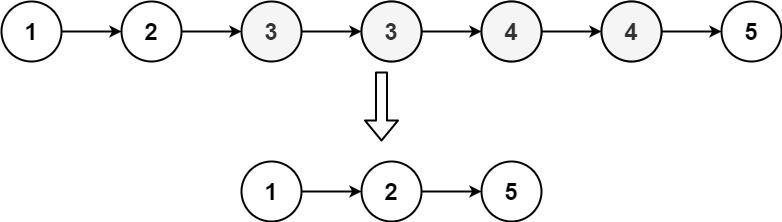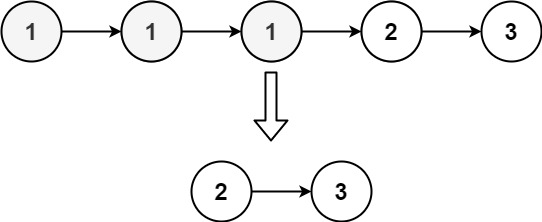## Algorithm

Problem Name: 82. Remove Duplicates from Sorted List II

Given the `head` of a sorted linked list, delete all nodes that have duplicate numbers, leaving only distinct numbers from the original list. Return the linked list sorted as well.

Example 1:```Input: head = [1,2,3,3,4,4,5]
Output: [1,2,5]
```

Example 2:```Input: head = [1,1,1,2,3]
Output: [2,3]
```

Constraints:

• The number of nodes in the list is in the range `[0, 300]`.
• `-100 <= Node.val <= 100`
• The list is guaranteed to be sorted in ascending order.

## Code Examples

### #1 Code Example with C Programming

```Code - C Programming```

``````
struct ListNode* deleteDuplicates(struct ListNode* head) {
struct ListNode *a, *b, *prev = NULL;
while (a) {
b = a->next;
if (!b || a->val != b->val) {
else prev->next = a;
prev = a;
} else {
do {  // skip all duplicated ones
b = b->next;
} while (b && b->val == a->val);
}
a = b;
}
if (prev) prev->next = NULL;
}
``````
Copy The Code &

Input

cmd

Output

cmd
[1,2,5]

### #2 Code Example with C++ Programming

```Code - C++ Programming```

``````
class Solution {
public:
ListNode res(0);
ListNode* pre = &res, *cur = head, *next = cur ? cur->next : NULL;
while(cur){
bool dup = false;
while(next && next->val == cur->val){
dup = true;
next = next->next;
}
if(dup){
cur = next;
next = next ? next->next : NULL;
pre->next = cur;
}
else{
pre = cur;
cur = next;
next = next ? next->next : NULL;
}
}
return res.next;
}
};
``````
Copy The Code &

Input

cmd

Output

cmd
[1,2,5]

### #3 Code Example with Java Programming

```Code - Java Programming```

``````
class Solution {
ListNode prev = null;
while (curr != null) {
if (curr.next != null && curr.next.val == curr.val) {
int currVal = curr.val;
while (curr != null && curr.val == currVal) {
curr = curr.next;
}
if (prev == null) {
} else {
prev.next = curr;
}
} else {
prev = curr;
curr = curr.next;
}
}
}
}
``````
Copy The Code &

Input

cmd

Output

cmd
[2,3]

### #4 Code Example with Javascript Programming

```Code - Javascript Programming```

``````
let dummy = new ListNode(undefined);
let prev = dummy;

while(curr) {
while(curr.next && curr.next.val === curr.val) {
curr = curr.next;
}
if(prev.next === curr) { // detect if it has deleted some elements
prev = prev.next;
curr = curr.next;
} else {
prev.next = curr.next;
curr = curr.next;
}
}

return dummy.next;
};
``````
Copy The Code &

Input

cmd

Output

cmd
[2,3]

### #5 Code Example with Python Programming

```Code - Python Programming```

``````
class Solution:
prev, prev_num = None, dummy_left
if prev and prev.val != head.val:
prev_num = prev
if prev and prev.val == head.val:
return dummy_left.next
``````
Copy The Code &

Input

cmd

Output

cmd
[1,2,5]

### #6 Code Example with C# Programming

```Code - C# Programming```

``````
namespace LeetCode
{
public class _082_RemoveDuplicatesFromSortedList2
{
{
var first = new ListNode(-1)
{
};

bool isDuplicate = false;
var prev = first;

for (var p = head; p != null && p.next != null; p = p.next)
{
if (!isDuplicate)
if (p.val == p.next.val)
isDuplicate = true;
else
prev = p;
else if (p.val != p.next.val)
{
isDuplicate = false;
prev.next = p.next;
}
}
if (isDuplicate)
{
prev.next = null;
}

return first.next;
}
}
}
``````
Copy The Code &

Input

cmd